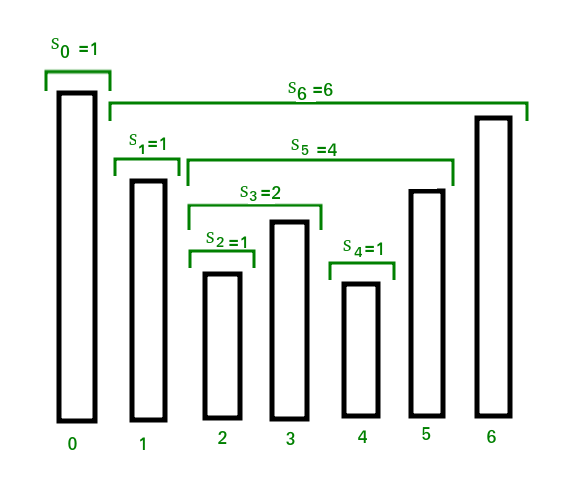The stock span problem is a financial problem where we have a series of n daily price quotes for a stock and we need to calculate span of stock’s price for all n days. The span Si of the stock’s price on a given day i is defined as the maximum number of consecutive days just before the given day, for which the price of the stock on the current day is less than or equal to its price on the given day. For example, if an array of 7 days prices is given as {100, 80, 60, 70, 60, 75, 85}, then the span values for corresponding 7 days are {1, 1, 1, 2, 1, 4, 6}METHOD 1

``````import org.junit.Test;

import java.util.Stack;

import static org.junit.Assert.assertArrayEquals;

/**
* Author     : Max
* Question   : https://www.geeksforgeeks.org/the-stock-span-problem/
* Complexity : time: O(n) ; space: O(n)
*/

public class StockSpanProblem {
static int[] calculateSpan(int[] arr) {
int n = arr.length;
Stack<Integer> s = new Stack<>();
int[] result = new int[n];

for (int i = 0; i < n; i++) {
int price = arr[i];
if (!s.isEmpty()) {
while (!s.isEmpty() && price >= arr[s.peek()]) {
s.pop();
}
}
result[i] = s.isEmpty() ? i + 1 : i - s.peek();
s.push(i);
}

return result;
}

@Test
public void test() {
int price[] = {10, 4, 5, 90, 120, 80};
assertArrayEquals(new int[]{1, 1, 2, 4, 5, 1}, calculateSpan(price));
}
}
``````